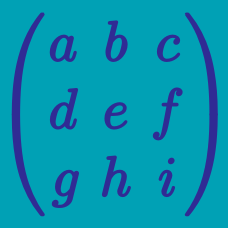Quantitative Finance

# Matrix Multiplication

Compute the following matrix multiplication: $\begin{pmatrix} 2 & 2 \\ 1 & 3 \end{pmatrix} \times \begin{pmatrix} 2 & 4 \\ 1 & 3 \end{pmatrix}.$

At an amusement park, tickets for the Pirate ship ride are $$5.00$ per adult and$$9.00$ per child. One sunny day, $558$ adults and $880$ children purchase tickets for this ride. The following day is rainy and only $219$ adults and $406$ children purchase tickets for this ride. By representing the number of rides in a matrix, calculate the total ticket sales (in dollars) for the ride over the two days.

Compute the following matrix multiplication: $\begin{pmatrix} 2 & 2 & 3 \\ 2 & 2 & 1 \end{pmatrix} \times \begin{pmatrix} 2 & 0 \\ 1 & 2 \\ 3 & 1 \end{pmatrix}.$

Find the value of $x+ y$ which satisfies the following equation: $\begin{pmatrix} 7 & 1 \\ 3 & 2 \end{pmatrix} \times \begin{pmatrix} x \\ y \end{pmatrix} = \begin{pmatrix} 2 \\ -51 \end{pmatrix}.$

Find the $3 \times 3$ matrix $A$ satisfying $A \times \begin{pmatrix} 1 \\ 1 \\ 0 \end{pmatrix} = \begin{pmatrix} 5 \\ 5 \\ 6 \end{pmatrix}, A \times \begin{pmatrix} 0 \\ 1 \\ 1 \end{pmatrix} = \begin{pmatrix} 5 \\ 3 \\ 3 \end{pmatrix}, A \times \begin{pmatrix} 1 \\ 0 \\ 1 \end{pmatrix} = \begin{pmatrix} 6 \\ 4 \\ 3 \end{pmatrix}.$

×